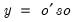Command Reference : Matrix Language Reference

 @qform
Syntax: @qform(s, o)
s: sym
o: vector, matrix, sym
Return: number, sym
Returns the quadratic form of a symmetric matrix s, with a vector or matrix object o.if o is a vector, the function returns a scalar
If o is a matrix, the function returns a sym
Examples
sym mom = @inner(@mnrnd(20, 4))
vector v1 = @mrnd(4)
scalar qf = @qform(@inverse(mom), v1)
generates a symmetric matrix MOM, then computes the quadratic form using the inverse of MOM, and the randomly generated vector V1.
matrix m1 = @mrnd(4, 5)
sym qfs = @qform(@inverse(mom), m1)
computes the matrix form of the quadratic form, returning a sym.
Cross-references